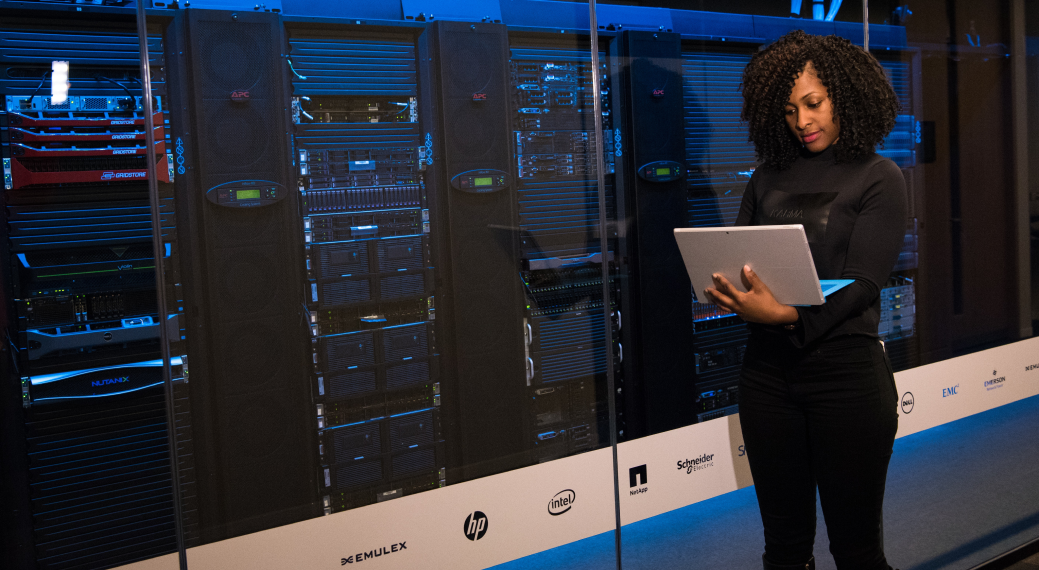We know what statistics is, but what about Machine Learning? It is the branch of Artificial Intelligence that seeks to understand the nature of data by means of automated data review for different tasks such as: value predictions, classification, clustering… Typically, this is as simple as taking the statistical linear model: Prediction = m · Data + c. And develop an algorithm that automatically calculates the coefficients m and c each time the number of data change.### Then, what is Deep Learning?

It is an extension of Machine Learning that seeks to achieve greater automation in data analysis and pattern recognition by imitating the way the human brain works (Neural Networks). It can offer solutions to models that conventional Machine Learning is not capable of understanding, in exchange for a notable increase in complexity and resource consumption that makes it difficult, among other things, to implement in real time.

So… Is it better to use Machine Learning or Deep Learning? Should we remain with basic statistics? The answer is: It Depends. All three options offer us useful and powerful tools that give excellent results. It is up to the analyst to decide which of these tools to use.

Thanks to these tools we can calculate the probability of a part being defective. We can calculate it at each Workstation where data is collected and thus avoid wasting resources on a part that is going to be defective.
Through this know-how, we have made great achievements such as reduce a reducing a reject rate of 12%, which generates a scrap cost of millions per year, to a 1.4% reject rate.

## Related Posts

The increase in digitization and data analysis in factories and its benefitsEnrique Soto

Software Engineer

Creating win-win relationships with our clientsInma Navarro

Data Analyst

The importance of data quality in data analysisIván Buhigas

Software Engineer